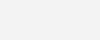# Using Serial-in-Parallel Out Shift RegisterSerial in Parallel Out Shift Register 74164

Here we use the IC 74164 an Eight Bit Serial-in-Parallel Out Shift register. The Data is given serially through one pin. To connect 64 LEDs we need eight 74164 chips. We are giving same clock to eight chips and different data input lines. Thus we need 8 Output pin for Data and 1 Output pin for Clock of the PIC Microcontroller. We can see that the pin number is reduced than in the previous method, but this method is a bit slower compared to the previous. The IC 74164 has the following pins.

• Data (A and B, these two pins are ANDed internally)
• Clock (CP)
• Chip Enable (MR – Here we don’t use this pin)

Here we connect the data input pin A of each chip to an Output pin of  PIC Microcontroller and B is tied to Vcc (LOGIC HIGH). The Clock pin (CP) of each chip is tied together and connected to another output pin of PIC Microcontroller. Thus we can easily control the 64 LEDs very easily.

## Circuit DiagramExpanding Output Pins of a PIC Microcontroller through Multiplexing Alternate Method

The following example MikroC Code works the above circuit as a Chaser.

## MikroC Code

```void main()
{
int j;
CMCON = 0x07; // To turn off comparators
ADCON1 = 0x06; // To turn off analog to digital converters
TRISB = 0x00;
TRISD = 0x00;
PORTD = 0x00;

do
{
PORTB = 0xFF;
for(j=0;j<8;j++)
{
PORTD.F7 = 1;
Delay_ms(50);
PORTD.F7 = 0;
PORTB = 0;
Delay_ms(200);
}
}while(1);
}```

Here you can download MikroC Code, Proteus Files, Circuit Diagrams etc..

Expanding Output Pins of a PIC Microcontroller